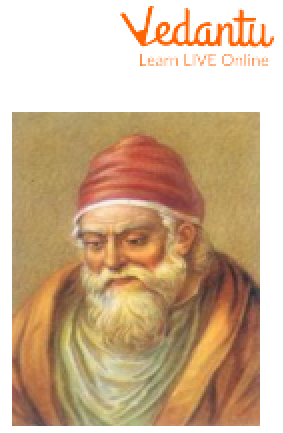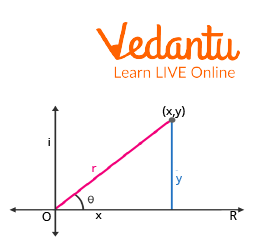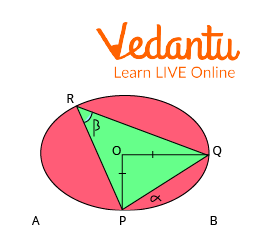Courses
Courses for Kids
Free study material
Free LIVE classes
More

# Difference between Axiom and TheoremLIVE
Join Vedantu’s FREE Mastercalss

## An Introduction to Theorems, Postulates, and Axioms

Axioms are mathematical statements that are assumed to be true by mathematicians but don’t have any logical proof.

Axiom Examples: $2+2\text{ }=\text{ }4$, $~3\text{ }x\text{ }3\text{ }=\text{ }9$ there is no logical proof for these statements, but they are true.

Theorems can be defined as those mathematical statements which are true and have a logical proof.

Theorem Examples: De Moivre’s Theorem, Alternate Segment Theorem, etc.

Postulates: In geometry, the mathematical statements that are assumed to be true by mathematicians don’t have any logical proof.

Postulates Examples: A line extends till infinity, an intersection of two planes is a line, etc.

### History of the MathematicianEuclid

Name: Euclid

Born: Mid-4th century BC

Field: Mathematics

Contribution: Euclid was the very first person to start discovering these axioms and theorems.

Nationality: Greek

## What is Theorem?

A theorem is a mathematical statement that is true and has very logical proof. It can either be for algebra or geometry, but the result of a theorem can always be proved. For example, De Moivre’s Theorem.

## Statement of De Moivre’s Theorem

According to the De Moivre Theorem, if we raise the power of a polar complex number by n, then it is equivalent to increasing the modulus to the same power and multiplying it by the argument raised to the same power. That means:

${{(\cos x+\sin x)}^{n}}=\cos (nx)+\sin (nx)$.

## Proof of De Moivre’s Theorem

Let us take a complex number $z=x+iy$.

This complex number can be written in polar form as $z=r(\cos \theta +i\sin \theta)$.

Here, $r=\sqrt{{{x}^{2}}+{{y}^{2}}}$ (𝛄 is called the modulus/absolute value of the complex number).

When we plot the complex number on the argand plane:Proof the De Moivre's Theorem

$\cos \theta =\dfrac{x}{r}$

$\sin \theta =\dfrac{y}{r}$

Now, let’s raise the complex number z to the power n.

$z=r(\cos \theta +i\sin \theta )$

${{z}^{n}}={{(r(\cos \theta +i\sin \theta ))}^{n}}$

${{z}^{n}}={{r}^{n}}{{(\cos \theta +i\sin \theta )}^{n}}$

Solving using the principle of mathematical induction:

For $n=1$

${{(\cos \theta +i\sin \theta )}^{1}}=\cos (1\theta )+\sin (1\theta )$

Assuming this to be true for $n=k$

${{(\cos \theta +i\sin \theta )}^{k}}=\cos (k\theta )+\sin (k\theta )$

Proving this to be true for $n=k+1$

${{(\cos \theta +i\sin \theta )}^{k+1}}={{(\cos \theta +i\sin \theta )}^{k}}.{{(\cos \theta +i\sin \theta )}^{1}}$

${{(\cos \theta +i\sin \theta )}^{k+1}}=(\cos (k\theta )+i\sin (k\theta )).(\cos \theta +i\sin \theta )$

${{(\cos \theta +i\sin \theta )}^{k+1}}=\cos (k\theta ).\cos (\theta )+i\cos (k\theta ).\sin \theta +i\sin (k\theta )\cos (\theta )-\sin (k\theta )\sin (\theta )$

${{(\cos \theta +i\sin \theta )}^{k+1}}=\cos (k\theta ).\cos (\theta )-\sin (k\theta )\sin (\theta )+i(\cos (k\theta ).\sin \theta +\sin (k\theta )\cos (\theta ))$

Using trigonometry formulae:

${{(\cos \theta +i\sin \theta )}^{k+1}}=\cos (k+1)\theta +i(\sin (k+1)\theta )$

Hence Proved.

## What are Axioms?

Those mathematical statements are assumed to be true by mathematicians, but they don’t have any logical proof. Let us take the example of Euclid’s axioms as examples of axioms:

• Things are equal to one another if they are equal to the same object.

• The wholes are equal if like items are added together.

• Equals can be subtracted from equals with equal results.

• Things are equivalent to one another if they occur simultaneously.

• The whole is superior to the parts.

## Application of De Moivre’s Theorem

De Moivre’s theorem can also be used to calculate the nth roots of a complex number:

Let a complex number be $z=\cos x+i\sin x$.

nth roots of z will be:

${{r}^{(\dfrac{1}{n})}}(\cos (\dfrac{x+2k\pi }{n})+i\sin (\dfrac{x+2k\pi }{n}))$

Here, k = 0,1,2……..(n-1)

## Important Points

• The value of n should always be an integer.

• The value of Ө will change if the complex number is not lying in the first quadrant.

• The nth root equation can also be used to calculate the roots of unity.

## Solved Examples

1. Name some theorems other than De Moivre’s theorem.

Ans: Names of some other theorems are listed below:

2. What is the alternate segment theorem?

Ans: For every circle, the angle created by the chord in the alternative segment equals the angle formed by the tangent via the point of contact of the tangent.Representation of Alternate Segment Theorem

Hence, according to the definition of the alternate segment theorem, $\angle \alpha =\angle \beta$

3. What is the basic proportionality theorem?

Ans: The basic Proportionality Theorem asserts that if a line is drawn parallel to any one side of a triangle and crosses the other two sides at two different positions, the line splits the other two sides in the same proportion.

## Important Points

• Any statement which does not have proof can’t be called an axiom. For any theorem to be called an axiom, it has to be valid universally.

• Once we have adopted a given proof system that is sound, and the axioms are all necessarily true, then only the theorems are true.

## Conclusion

The difference between theorems, axioms, and postulates is a very important concept in the world of Mathematics. The term ‘Axioms’ is related to the whole branch of Mathematics while the term ‘Postulate’ should only be used for geometry.

Last updated date: 19th Sep 2023
Total views: 94.5k
Views today: 1.94k

## FAQs on Difference between Axiom and Theorem

1. What is Euclidean geometry?

The Greek words "geo," which means "earth," and "metria," which means "measuring," are combined to form the English word "geometry." The study of geometrical shapes, whether two-dimensional or three-dimensional, and their relationships in terms of points, lines, and planes is known as Euclidean geometry.

Euclid was a very great mathematician of his era, and his theories have helped a lot of scientists to discover their theories. Several different axioms and theorems make up Euclid's geometry. Plane Geometry and Solid Geometry are the two main topics covered by Euclid's geometry.

2. Why is Euclid's geometry not considered sound these days?

Although Euclid's Axiom formulation is bright and clear, it does not meet the requirements of current axiom systems. First, the reader is requested to "idealise" the basic concepts of point, line, angle, and circle, which are taken from everyday life and have no size, thickness, or end. Modern axiom systems, like set theory, avoid attempting to define the meaning of its key ideas (set, membership). Instead, they explain how the central idea(s) act and they have proven to be more accurate than Euclid's Postulates.

3. What are conjectures in Mathematics?

A hypothesis in Mathematics is a conclusion, or a proposition made tentatively without supporting evidence. Some conjectures, like the Riemann Hypothesis, which is still an assumption, and Fermat's Last Theorem, which Andrew Wiles proved in 1995, have influenced much of the history of Mathematics as new branches of Mathematics are created to prove them. An example of conjectures is Linear Pair Conjecture which talks about adjacent angles formed by two intersecting lines and states that their sum is supplementary.